高斯低通滤波器_高斯低通滤波器公式 - CSDN

• 高斯低通滤波有频域和时域；在用作光滑图像上，可以卷积，可以相乘； 高斯滤波器宽度(决定着平滑程度)是由参数σ表征的，而且σ和平滑程度的...2D高斯低通滤波器来定义： 其中，x和y是坐标，是控制高斯核形状...
高斯低通滤波有频域和时域；在用作光滑图像上，可以卷积，可以相乘；
高斯滤波器宽度(决定着平滑程度)是由参数σ表征的，而且σ和平滑程度的关系是非常简单的。σ越大，高斯滤波器的频带就越宽，平滑程度就越好。通过调节平滑程度参数σ，可在图像特征过分模糊(过平滑)与平滑图像中由于噪声和细纹理所引起的过多的不希望突变量(欠平滑)之间取得折衷。
2D高斯低通滤波器来定义：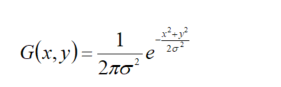其中，x和y是坐标，是控制高斯核形状的方差（本文取1）。
%% 频域滤波函数lpfilter
通过查阅文献，百度网页：https://blog.csdn.net/weixin_39124778/article/details/78411314
https://blog.csdn.net/cjsh_123456/article/details/79342300
论文：改善数字图像质量的滤波方法研究_朱美华


展开全文• 低通滤波器  1.理想的低通滤波器  其中，D0表示通带的半径。D(u,v)的计算方式也就是两点间的距离，很简单就能得到。  使用低通滤波器所得到的结果如下所示。低通滤波器滤除了高频...

低通滤波器

1.理想的低通滤波器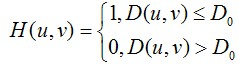其中，D0表示通带的半径。D(u,v)的计算方式也就是两点间的距离，很简单就能得到。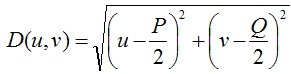使用低通滤波器所得到的结果如下所示。低通滤波器滤除了高频成分，所以使得图像模糊。由于理想低通滤波器的过度特性过于急峻，所以会产生了振铃现象。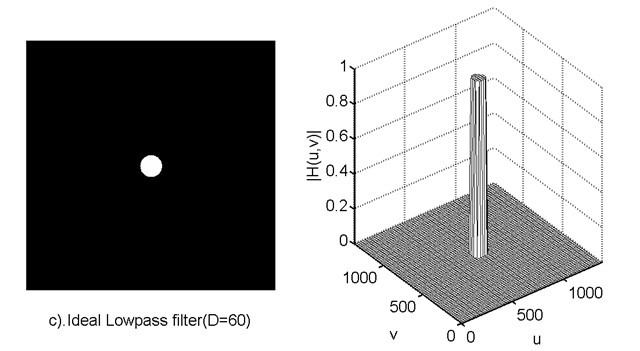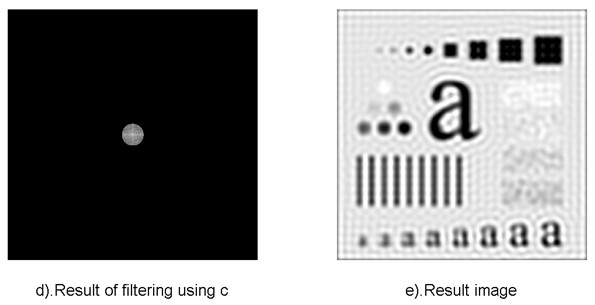2.巴特沃斯低通滤波器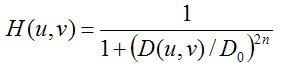同样的，D0表示通带的半径，n表示的是巴特沃斯滤波器的次数。随着次数的增加，振铃现象会越来越明显。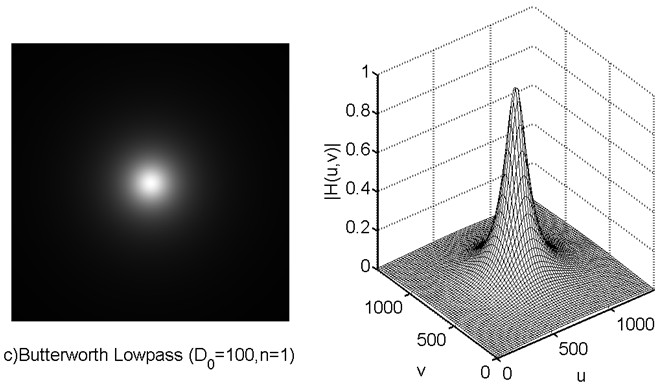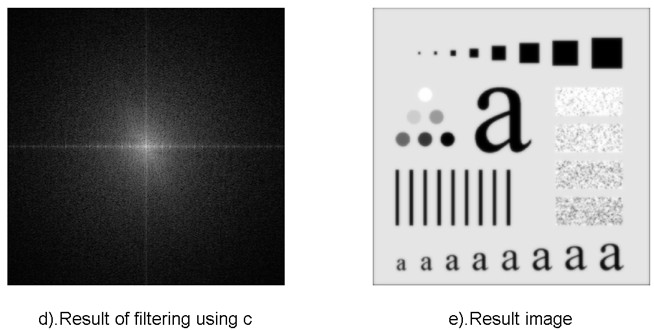3.高斯低通滤波器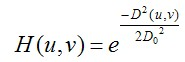D0表示通带的半径。高斯滤波器的过度特性非常平坦，因此是不会产生振铃现象的。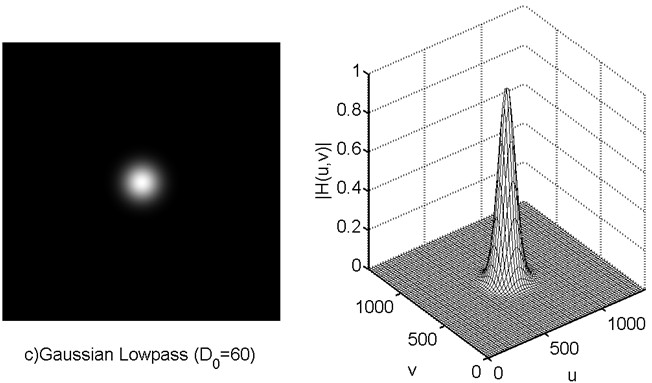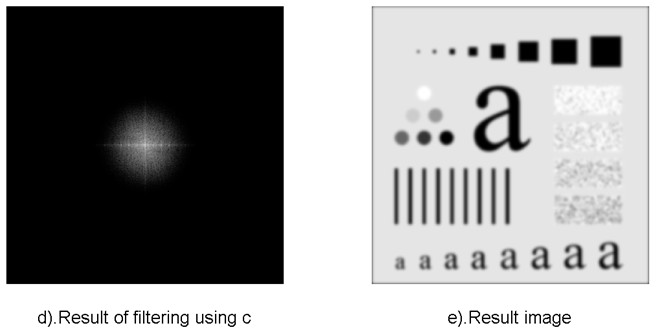转载出处： http://blog.csdn.net/zhoufan900428/article/details/17194289


展开全文•  1.傅里叶变换与频域 ...比如，使用低通滤波可以将图像模糊，也有些许降噪的作用。这些都是在空间域内进行的滤波处理，这个处理主要是依靠卷积来进行计算的。首先，从连续的一维卷积入手，如下所示。
转自http://blog.csdn.net/zhoufan900428/article/details/17194289

1.傅里叶变换与频域

在之前的文中，我们已经进行过一些基本的图像处理。比如，使用低通滤波可以将图像模糊，也有些许降噪的作用。这些都是在空间域内进行的滤波处理，这个处理主要是依靠卷积来进行计算的。首先，从连续的一维卷积入手，如下所示。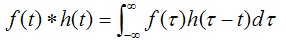将上式进行傅里叶变换，可以得到如下结果。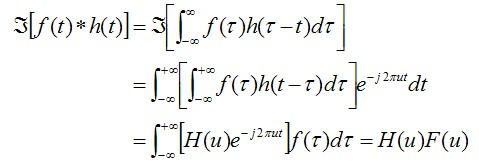从这个式子，我们可以得到一个重要的结论。也就是，函数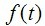与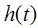卷积的傅里叶变换所得到的结果，是函数与的傅里叶变换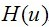与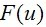的乘积。再将其总结得简单易懂一些，有如下结论。在将其扩展到二维的形况下，假设尺寸为MxN的图像，如下关系是成立的。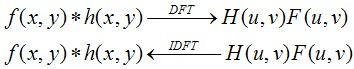其实到这，基本的原理就明了的。我们所看到的图像，均为空间域内的表现形式，我们无法辨识出频域内的图像。要进行频域内的滤波器处理，首先就需要进行傅里叶变换，然后直接进行滤波处理，最后再用反傅里叶变换倒回到空间域内。

到此，已经可以开始空间域内的滤波处理了。但是，还有一点需要注意的地方。使用某个一维信号来举例子，一维信号的傅里叶变换是以2π为周期的函数。所以，我们常常使用的范围[-π,π]来表示这个信号的傅里叶变换，如下所示。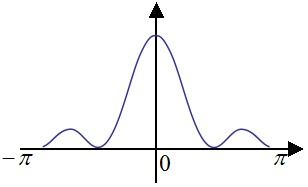这样做的好处是，靠近0的成分就是低频，靠近-π与π的成分就表示高频。而对于图像而言，在Matlab中，我们使用fft2()这个函数来求取图像的傅里叶变换。

[plain] view
plain copyg = fft2(f);

上面这个代码求取的，其实是范围[0,π]内的傅里叶变换。为了方便理解，下图画出了本行代码所求取的图像的傅里叶变换的范围（右）和与其等效的一维傅里叶变换的范围（左）。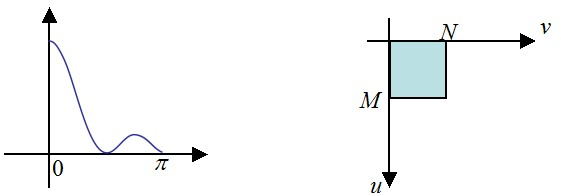很显然，这并不是希望的范围，下面这个代码可以求取[0,2π]内的傅里叶变换。

[plain] view
plain copyP = 2*M;
Q = 2*N;
F = fft2(f,P,Q);

下图画出了本行代码所求取的图像的傅里叶变换的范围（右）和与其等效的一维傅里叶变换的范围（左）。所得到的图像F(u,v)的尺寸为PxQ。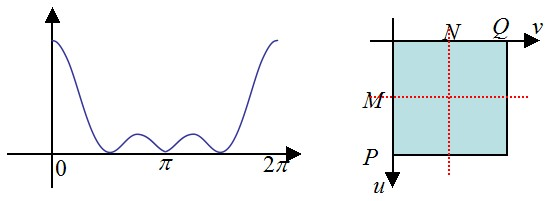我们需要对其移动一下，如下图所示，我们需要的是粉色范围的区域。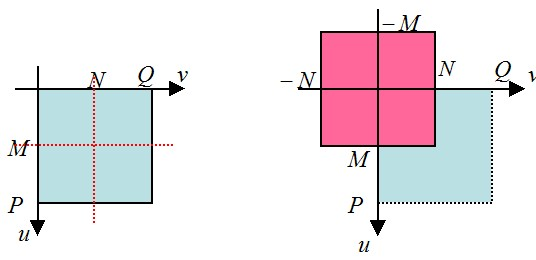下面，从数学上分析一下，如何获得这个部分的频谱。对于傅里叶变换，有如下性质。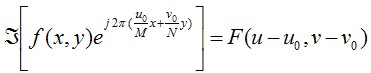这个特性称为平移特性，粉色部分的频谱，将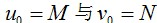带入上式，我们可以得到如下式子。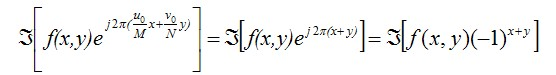为次，我们已经得到了粉色范围的频谱。越靠近傅里叶频谱图像中间的成分，代表了低频成分。其Matlab代码如下所示。

[plain] view
plain copy[M,N] = size(f);
P = 2*M;
Q = 2*N;
fc = zeros(M,N);

for x = 1:1:M
for y = 1:1:N
fc(x,y) = f(x,y) * (-1)^(x+y);
end
end

F = fft2(fc,P,Q);

代码所得到的结果，如下图所示。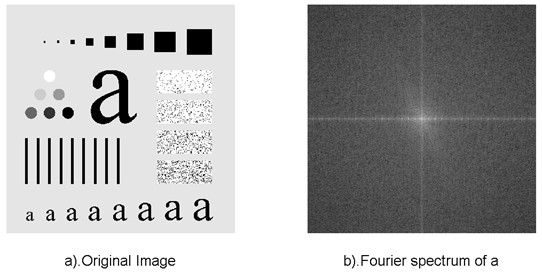接下来，我们总结一下频域滤波的步骤：

①：先将图像做频域内的水平移动，然后求原图像f(x,y)的DFT，得到其图像的傅里叶谱F(u,v)。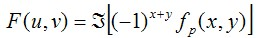②：与频域滤波器做乘积，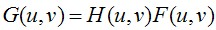③：求取G(u,v)的IDFT，然后再将图像做频域内的水平移动（移动回去），其结果可能存在寄生的虚数，此时忽略即可。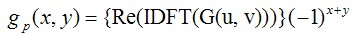④：这里使用ifft2函数进行IDFT变换，得到的图像的尺寸为PxQ。切取左上角的MxN的图像，就能得到结果了。

2.低通滤波器
2.1理想的低通滤波器其中，D0表示通带的半径。D(u,v)的计算方式也就是两点间的距离，很简单就能得到。使用低通滤波器所得到的结果如下所示。低通滤波器滤除了高频成分，所以使得图像模糊。由于理想低通滤波器的过度特性过于急峻，所以会产生了振铃现象。2.2巴特沃斯低通滤波器同样的，D0表示通带的半径，n表示的是巴特沃斯滤波器的次数。随着次数的增加，振铃现象会越来越明显。2.3高斯低通滤波器D0表示通带的半径。高斯滤波器的过度特性非常平坦，因此是不会产生振铃现象的。3.实现代码

1
2
3
4
5
6
7
8
9
10
11
12
13
14
15
16
17
18
19
20
21
22
23
24
25
26
27
28
29
30
31
32
33
34
35
36
37
38
39
40
41
42
43
44
45
46
47
48
49
50
51
52
53
54
55
56
57
58
59
60
61
62
63
64
65
66
67
68
69
70
71
72
73
74
75
76
77
78
79
80
81
82
83
84
85
86
87
88
89
90
91
92
93
94
95
96
97
98
99
100

close all;clear all;
%% ---------Butterworth Lowpass Filters (Fre. Domain)------------f = imread('characters_test_pattern.tif');f = mat2gray(f,[0 255]);
[M,N] = size(f);P = 2*M;Q = 2*N;fc = zeros(M,N);
for x = 1:1:M    for y = 1:1:N        fc(x,y) = f(x,y) * (-1)^(x+y);    endend
F = fft2(fc,P,Q);
H_1 = zeros(P,Q);H_2 = zeros(P,Q);
for x = (-P/2):1:(P/2)-1     for y = (-Q/2):1:(Q/2)-1        D = (x^2 + y^2)^(0.5);        D_0 = 100;        H_1(x+(P/2)+1,y+(Q/2)+1) = 1/(1+(D/D_0)^2);           H_2(x+(P/2)+1,y+(Q/2)+1) = 1/(1+(D/D_0)^6);     endend

G_1 = H_1 .* F;G_2 = H_2 .* F;
g_1 = real(ifft2(G_1));g_1 = g_1(1:1:M,1:1:N);
g_2 = real(ifft2(G_2));g_2 = g_2(1:1:M,1:1:N);
for x = 1:1:M    for y = 1:1:N        g_1(x,y) = g_1(x,y) * (-1)^(x+y);        g_2(x,y) = g_2(x,y) * (-1)^(x+y);    endend
%% -----show-------figure();subplot(1,2,1);imshow(f,[0 1]);xlabel('a).Original Image');
subplot(1,2,2);imshow(log(1 + abs(F)),[ ]);xlabel('b).Fourier spectrum of a');
figure();subplot(1,2,1);imshow(H_1,[0 1]);xlabel('c)Butterworth Lowpass (D_{0}=100,n=1)');
subplot(1,2,2);h = mesh(1:20:P,1:20:Q,H_1(1:20:P,1:20:Q));set(h,'EdgeColor','k');axis([0 P 0 Q 0 1]);xlabel('u');ylabel('v');zlabel('|H(u,v)|');
figure();subplot(1,2,1);imshow(log(1 + abs(G_1)),[ ]);xlabel('d).Result of filtering using c');
subplot(1,2,2);imshow(g_1,[0 1]);xlabel('e).Result image');
figure();subplot(1,2,1);imshow(H_2,[0 1]);xlabel('f).Butterworth Lowpass (D_{0}=100,n=3)');
subplot(1,2,2);h = mesh(1:20:P,1:20:Q,H_2(1:20:P,1:20:Q));set(h,'EdgeColor','k');axis([0 P 0 Q 0 1]);xlabel('u');ylabel('v');zlabel('|H(u,v)|');
figure();subplot(1,2,1);imshow(log(1 + abs(G_2)),[ ]);xlabel('g).Result of filtering using e');
subplot(1,2,2);imshow(g_2,[0 1]);xlabel('h).Result image');

来自CODE的代码片
Butterworth_Lowpass_Filters.m

1
2
3
4
5
6
7
8
9
10
11
12
13
14
15
16
17
18
19
20
21
22
23
24
25
26
27
28
29
30
31
32
33
34
35
36
37
38
39
40
41
42
43
44
45
46
47
48
49
50
51
52
53
54
55
56
57
58
59
60
61
62
63
64
65
66
67
68
69
70
71
72
73
74
75
76
77
78
79
80
81
82
83
84
85
86
87
88
89
90
91
92
93
94
95
96
97
98
99
100
101
102
103

close all;clear all;clc;%% ---------Gaussian Lowpass Filters (Fre. Domain)------------f = imread('characters_test_pattern.tif');f = mat2gray(f,[0 255]);
[M,N] = size(f);P = 2*M;Q = 2*N;fc = zeros(M,N);
for x = 1:1:M    for y = 1:1:N        fc(x,y) = f(x,y) * (-1)^(x+y);    endend
F = fft2(fc,P,Q);
H_1 = zeros(P,Q);H_2 = zeros(P,Q);
for x = (-P/2):1:(P/2)-1     for y = (-Q/2):1:(Q/2)-1        D = (x^2 + y^2)^(0.5);        D_0 = 60;        H_1(x+(P/2)+1,y+(Q/2)+1) = exp(-(D*D)/(2*D_0*D_0));           D_0 = 160;        H_2(x+(P/2)+1,y+(Q/2)+1) = exp(-(D*D)/(2*D_0*D_0));     endend
G_1 = H_1 .* F;G_2 = H_2 .* F;
g_1 = real(ifft2(G_1));g_1 = g_1(1:1:M,1:1:N);
g_2 = real(ifft2(G_2));g_2 = g_2(1:1:M,1:1:N);
for x = 1:1:M    for y = 1:1:N        g_1(x,y) = g_1(x,y) * (-1)^(x+y);        g_2(x,y) = g_2(x,y) * (-1)^(x+y);    endend

%% -----show-------close all;
figure();subplot(1,2,1);imshow(f,[0 1]);xlabel('a).Original Image');
subplot(1,2,2);imshow(log(1 + abs(F)),[ ]);xlabel('b).Fourier spectrum of a');
figure();subplot(1,2,1);imshow(H_1,[0 1]);xlabel('c)Gaussian Lowpass (D_{0}=60)');
subplot(1,2,2);h = mesh(1:20:P,1:20:Q,H_1(1:20:P,1:20:Q));set(h,'EdgeColor','k');axis([0 P 0 Q 0 1]);xlabel('u');ylabel('v');zlabel('|H(u,v)|');
figure();subplot(1,2,1);imshow(log(1 + abs(G_1)),[ ]);xlabel('d).Result of filtering using c');
subplot(1,2,2);imshow(g_1,[0 1]);xlabel('e).Result image');
figure();subplot(1,2,1);imshow(H_2,[0 1]);xlabel('f).Gaussian Lowpass (D_{0}=160)');
subplot(1,2,2);h = mesh(1:20:P,1:20:Q,H_2(1:20:P,1:20:Q));set(h,'EdgeColor','k');axis([0 P 0 Q 0 1]);xlabel('u');ylabel('v');zlabel('|H(u,v)|');
figure();subplot(1,2,1);imshow(log(1 + abs(G_2)),[ ]);xlabel('g).Result of filtering using e');
subplot(1,2,2);imshow(g_2,[0 1]);xlabel('h).Result image');

来自CODE的代码片
Gaussian_Lowpass_Filters.m

1
2
3
4
5
6
7
8
9
10
11
12
13
14
15
16
17
18
19
20
21
22
23
24
25
26
27
28
29
30
31
32
33
34
35
36
37
38
39
40
41
42
43
44
45
46
47
48
49
50
51
52
53
54
55
56
57
58
59
60
61
62
63
64
65
66
67
68
69
70
71
72
73
74
75
76
77
78
79
80
81
82
83
84
85
86
87
88
89
90
91
92
93
94
95
96
97
98

close all;clear all;
%% ---------Ideal Lowpass Filters (Fre. Domain)------------f = imread('characters_test_pattern.tif');f = mat2gray(f,[0 255]);
[M,N] = size(f);P = 2*M;Q = 2*N;fc = zeros(M,N);
for x = 1:1:M    for y = 1:1:N        fc(x,y) = f(x,y) * (-1)^(x+y);    endend
F = fft2(fc,P,Q);
H_1 = zeros(P,Q);H_2 = zeros(P,Q);
for x = (-P/2):1:(P/2)-1     for y = (-Q/2):1:(Q/2)-1        D = (x^2 + y^2)^(0.5);        if(D <= 60)  H_1(x+(P/2)+1,y+(Q/2)+1) = 1; end            if(D <= 160)  H_2(x+(P/2)+1,y+(Q/2)+1) = 1; end     endend
G_1 = H_1 .* F;G_2 = H_2 .* F;
g_1 = real(ifft2(G_1));g_1 = g_1(1:1:M,1:1:N);
g_2 = real(ifft2(G_2));g_2 = g_2(1:1:M,1:1:N);
for x = 1:1:M    for y = 1:1:N        g_1(x,y) = g_1(x,y) * (-1)^(x+y);        g_2(x,y) = g_2(x,y) * (-1)^(x+y);    endend
%% -----show-------figure();subplot(1,2,1);imshow(f,[0 1]);xlabel('a).Original Image');
subplot(1,2,2);imshow(log(1 + abs(F)),[ ]);xlabel('b).Fourier spectrum of a');
figure();subplot(1,2,1);imshow(H_1,[0 1]);xlabel('c).Ideal Lowpass filter(D=60)');
subplot(1,2,2);h = mesh(1:20:P,1:20:Q,H_1(1:20:P,1:20:Q));set(h,'EdgeColor','k');axis([0 P 0 Q 0 1]);xlabel('u');ylabel('v');zlabel('|H(u,v)|');
figure();subplot(1,2,1);imshow(log(1 + abs(G_1)),[ ]);xlabel('d).Result of filtering using c');
subplot(1,2,2);imshow(g_1,[0 1]);xlabel('e).Result image');
figure();subplot(1,2,1);imshow(H_2,[0 1]);xlabel('f).Ideal Lowpass filter(D=160)');
subplot(1,2,2);h = mesh(1:20:P,1:20:Q,H_2(1:20:P,1:20:Q));set(h,'EdgeColor','k');axis([0 P 0 Q 0 1]);xlabel('u');ylabel('v');zlabel('|H(u,v)|');
figure();subplot(1,2,1);imshow(log(1 + abs(G_2)),[ ]);xlabel('g).Result of filtering using e');
subplot(1,2,2);imshow(g_2,[0 1]);xlabel('h).Result image');


展开全文图像处理 傅里叶变换 低通滤波器
• 高斯低通滤波器其原理就是RC电路具有使低频信号较易通过而抑制较高频率信号的作用，可以有效地去除服从正态分布的噪声。
• 低通滤波器  1.理想的低通滤波器  其中，D0表示通带的半径。D(u,v)的计算方式也就是两点间的距离，很简单就能得到。  使用低通滤波器所得到的结果如下所示。低通滤波器滤除了高频成分，...
低通滤波器

1.理想的低通滤波器其中，D0表示通带的半径。D(u,v)的计算方式也就是两点间的距离，很简单就能得到。使用低通滤波器所得到的结果如下所示。低通滤波器滤除了高频成分，所以使得图像模糊。由于理想低通滤波器的过度特性过于急峻，所以会产生了振铃现象。2.巴特沃斯低通滤波器同样的，D0表示通带的半径，n表示的是巴特沃斯滤波器的次数。随着次数的增加，振铃现象会越来越明显。3.高斯低通滤波器D0表示通带的半径。高斯滤波器的过度特性非常平坦，因此是不会产生振铃现象的。展开全文• ## 高斯函数及高斯滤波器

万次阅读 多人点赞 2019-07-11 11:37:24
1 一维高斯分布 1.1 一维高斯分布的定义 若连续型随机变量X的概率密度为： 其中，为常数，则称X服从参数为，的正态分布或高斯分布，记为 1.2 一维高斯分布的曲线 横轴表示可能的取值x，竖轴表示...
• （1）自定义一个图像的频率域高斯低通滤波... 自定义的高斯低通滤波器： %pho参数为输入图像的路径 %D0参数为截止频率 function im2 = myGLPF(pho,D0) ima = imread(pho); %读取输入图像 %得到高斯低通滤波器 [r高斯低通滤波
• 一、简介我们知道，在一幅图像中，其低频成分对应者图像变化缓慢的部分，对应着图像大致的相貌和轮廓。而其高频成分则对应着图像变化剧烈的部分，对应着图像的细节（图像的噪声也属于高频成分）。...理想低通滤波器...matlab编程
• 版权声明：<–本博客所有内容均为自己在学习工作中的总结、摘录等-- --转载请注明出处-- --如有侵权请联系删除–> https://blog.csdn.net/ai359005521/article/details/85068305 ...
• 高斯低通滤波器 频域锐化滤波器 理想高通滤波器 巴特沃斯高通滤波器 高斯高通滤波器 频域拉普拉斯算子 高频提升滤波 同态滤波器 低通滤波器通过过滤掉频域的高频成分达到平滑目的。 滤波的基本模型G(u,v) = H(u,v)F...opencv 计算机视觉 matlab 信号处理
• 我有一个关于高斯低通滤波器的问题， 在许多的博客上（同样的情况在matlab的fspecial函数中），产生空间域滤波器 使用的公式都是A*exp(-u/(2*sigma^2))，之后再进行了归一化。但是这个公式不是高斯函数在频域域中的...matlab 数字图像处理 高斯滤波
• 1.高斯滤波器综述  高斯滤波器是一类根据高斯函数的形状来选择权值的线性平滑滤波器高斯平滑滤波器对于抑制服从正态分布的噪声非常有效。一维零均值高斯函数为：  g(x)=exp( -x^2/(2 sigma^2) 其中，...
• ## 频率域滤波器

千次阅读 2016-04-21 16:27:29
从滤波器处理效果的尖锐程度，可以将他们分为三种类型：理想滤波器、...理想低通滤波器： 理想高通滤波器： 理想带阻滤波器： 这里： 巴特沃斯低通滤波器： 巴特沃斯高通滤波器： 巴特沃斯带阻滤波器：
• 一、程序代码及注释1、myGLPF函数%pho参数为输入图像的路径... %读取输入图像 %得到高斯低通滤波器[r,c] = size(ima); %获取输入图像的行和列D = zeros(r,c); %D(u,v)是距频率矩形中心的距离for i=1:r for j=1:c ...数字图像处理
• OpenCV 学习（几种基本的低通滤波）对图像进行滤波处理是图像处理中最常见的一种操作类型。而这其中低通滤波（也可以叫做平滑）有事各种滤波处理中最常用的。这里就简单写写 OpenCV 中提供的几种低通滤波方法。均值...图像处理 opencv
• 本篇文章是超级简单的matlab进行图像滤波的程序，采用常用的频域滤波器进行简单的滤波操作，当然有时候空域效果较好。matlab 图像处理
• ## 常见低通滤波器

千次阅读 2018-03-29 17:04:50
转载自：https://blog.csdn.net/tianrolin/article/details/44084317 ... 1.理想的低通滤波器 其中，D0表示通带的半径。D(u,v)的计算方式也就是两点间的距离，很简单就能得到。 使用低通滤波器所得到的结...
• 二：空域滤波器如均值滤波器，中值滤波器，低通滤波器高斯滤波，双边滤波，引导滤波。  频域滤波器如小波变换，傅里叶变换，余弦变换，形态学滤波（通过膨胀和腐蚀等形态学进行去噪）。 三：应用 均值，中值，低...算法
• 高通滤波，低通滤波是按频率划分的。...那么保留高频就是高通滤波器（边缘提取），保留低频就是低通录波器（图像平滑）。 傅里叶变换将图像转换成幅值谱(magnitude_spectrum)：按频率从小到大由中心向四周高通滤波 低通滤波
• 低通滤波器 本文主要介绍三种常见低通滤波器的实现方法及其透视图 理想低通滤波器（ILPF） 理想低通滤波器有下面的函数确定： H(u,v)={1D(u,v)≤D00公式3条件3 H(u,v)=\begin{cases} 1 &amp; D(u,v) \leq D_0 \\...matlab 低通滤波器 滤波器实现
...